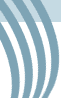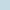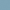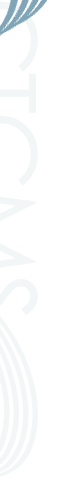# MassSpectator online calculator

MassSpectator is a mathematical method that locates, and calculates the area beneath, peaks from mass spectra using only reproducible mathematical operations and no user-selected parameters. It makes no assumptions about peak shape and requires no smoothing or preprocessing of the data. However, to facilitate this approach the method requires a background spectrum to be taken in order to determine inherent instrument noise. The background spectrum is taken under the same conditions as the data with the only difference being the absence of analyte.

The algorithm is based on a time-series segmentation routine that reduces the data set to groups of three strategic points where each group defines the beginning, center, and ending of each peak located. The peak areas are found from the strategic points using a simple polygonal area calculation routine. Peaks with statistically insignificant height or area are then discarded. The output file format contains one line per peak found. Each line consists of seven entries: the peak beginning x and y coordinates, the peak center x and y coordinates, the peak ending x and y coordinates and the area (2+2+2+1=7 entries per line).

The input files must be simple ASCII text files of two columns: one for mass and one for ion intensity. They can be tab, space, or comma delimited and must be of the same length. The spacing between mass values does not need to be equal (i.e. time-of-flight data converted to mass with its consequent square-root point spacing is OK); however, the mass values need to be monotonically increasing. Be sure to use a sufficient number of significant digits in the ion intensity. Some software packages will default to a number of significant digits that is too small for accurate calculation of background noise. Six significant figures should suffice; eight would be better.

MassSpectator is a collaborative project between the Polymers Division of the Materials Science and Engineering Laboratory and the Mathematical and Computational Sciences Division of the Information Technology Laboratory, both of NIST.

Details of this method can be found in the following two papers:

## Run a calculationNIST is an agency of the U.S. Commerce Department's Technology Administration